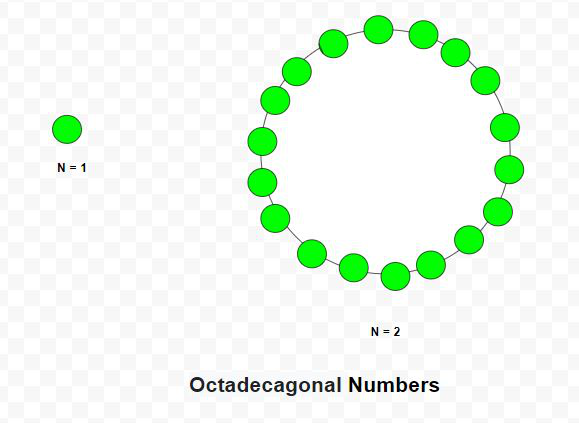Given a number N, the task is to find the Nth Octadecagonal number.

An Octadecagonal number is a class of figurate numbers. It has 18 – sided polygon called Octadecagon. The Nth Octadecagonal number count’s the eighteen number of dots and all other dots are surrounding with a common sharing corner and make a pattern. The first few Octadecagonal numbers are 1, 18, 51, 100, 165, 246, 343 …

Examples:

Input: N = 2
Output: 18
Explanation:
The second octadecagonal number is 18.

Input: N = 6
Output: 246

## Recommended: Please try your approach on {IDE} first, before moving on to the solution.Approach: The Nth Octadecagonal number is given by the formula:

``````

Below is the implementation of the above approach:

## C++

 `// C++ program to find Nth ` `// Octadecagonal number ` ` `  `#include ` `using` `namespace` `std; ` ` `  `// Function to find N-th ` `// Octadecagonal number ` `int` `Octadecagonal_num(``int` `n) ` `{ ` `    ``// Formula to calculate nth ` `    ``// Octadecagonal number ` `    ``return` `(16 * n * n - 14 * n) / 2; ` `} ` ` `  `// Driver code ` `int` `main() ` `{ ` `    ``int` `n = 3; ` `    ``cout << Octadecagonal_num(n) << endl; ` ` `  `    ``n = 10; ` `    ``cout << Octadecagonal_num(n) << endl; ` ` `  `    ``return` `0; ` `} `

## Java

 `// Java program to find Nth ` `// octadecagonal number ` `import` `java.io.*;  ` `import` `java.util.*;  ` ` `  `class` `GFG{  ` `     `  `// Function to find N-th ` `// octadecagonal number ` `static` `int` `Octadecagonal_num(``int` `n) ` `{ ` `         `  `    ``// Formula to calculate nth ` `    ``// octadecagonal number ` `    ``return` `(``16` `* n * n - ``14` `* n) / ``2``; ` `} ` `     `  `// Driver code  ` `public` `static` `void` `main(String[] args)  ` `{  ` `    ``int` `n = ``3``; ` `    ``System.out.println(Octadecagonal_num(n)); ` `     `  `    ``n = ``10``; ` `    ``System.out.println(Octadecagonal_num(n)); ` `}  ` `}  ` ` `  `// This code is contributed by coder001 `

## Python3

 `# Python3 program to find Nth  ` `# octadecagonal number ` ` `  `# Function to find N-th  ` `# octadecagonal number ` `def` `Octadecagonal_num(n): ` `     `  `    ``# Formula to calculate nth  ` `    ``# octadecagonal number  ` `    ``return` `(``16` `*` `n ``*` `n ``-` `14` `*` `n) ``/` `2` ` `  `# Driver code     ` `n ``=` `3` `print``(``int``(Octadecagonal_num(n)))  ` ` `  `n ``=` `10` `print``(``int``(Octadecagonal_num(n)))  ` ` `  `# This code is contributed by divyeshrabadiya07      `

## C#

 `// C# program to find Nth ` `// octadecagonal number ` `using` `System;  ` ` `  `class` `GFG{  ` `     `  `// Function to find N-th ` `// octadecagonal number ` `static` `int` `Octadecagonal_num(``int` `n) ` `{ ` `         `  `    ``// Formula to calculate nth ` `    ``// octadecagonal number ` `    ``return` `(16 * n * n - 14 * n) / 2; ` `} ` `     `  `// Driver code  ` `public` `static` `void` `Main(``string``[] args)  ` `{  ` `    ``int` `n = 3; ` `    ``Console.Write(Octadecagonal_num(n) + ``"\n"``); ` `     `  `    ``n = 10; ` `    ``Console.Write(Octadecagonal_num(n) + ``"\n"``); ` `}  ` `}  ` ` `  `// This code is contributed by rutvik_56 `

Output:

```51
730
```

Don’t stop now and take your learning to the next level. Learn all the important concepts of Data Structures and Algorithms with the help of the most trusted course: DSA Self Paced. Become industry ready at a student-friendly price.

My Personal Notes arrow_drop_upCheck out this Author's contributed articles.

If you like GeeksforGeeks and would like to contribute, you can also write an article using contribute.geeksforgeeks.org or mail your article to contribute@geeksforgeeks.org. See your article appearing on the GeeksforGeeks main page and help other Geeks.

Please Improve this article if you find anything incorrect by clicking on the "Improve Article" button below.

Article Tags :
Practice Tags :

Be the First to upvote.

Please write to us at contribute@geeksforgeeks.org to report any issue with the above content.